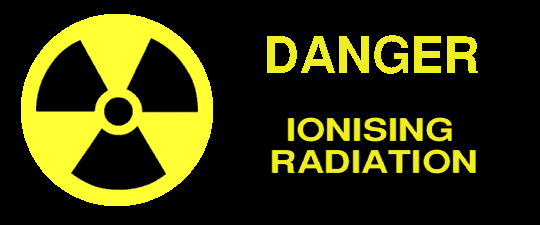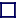BECQUERAL, (Bq)
Becquerals are a count of the number of nuclear disintegrations per second. According to the Radioactive Substances Act of 1983, anything containing more than 400 Bq/kg must be regarded as radioactive. American laws are much stricter. 1 becqueral=2.703 × 10-11 curies.

CURIE, (Ci)
One Curie is the radioactivity of 1 gm of radium, and is the equivalent of 37 billion becquerals.

The energy deposited in the body by radiation, the absorbed dose. When one kilogram of the body absorbs 0.01 joules of energy, that is one rad. 1 rad=0.01 Gray = 0.01 Joules per kilogram = 2.388 × 10-6 cal per gram = 6.242 × 1013eV per gram. The rad replaced the roentgen in 1956. 1000 rads = 1100 roentgens (approximately, depending upon the material exposed).

SIEVERT, (Sv)
A unit of gamma ray dose. Defined as the dose of radiation delivered in one hour at a distance of one centimetre from a point source of 1 mg of Radium contained in a platinum vessel 0.5 mm thick. There is a fundamental difference between the actual dose of ionizing radiation absorbed D (measured in grays) and the equivalent dose E (measured in siemens), both of which are measured in joules per kilogram, and it was to avoid the great harm to patients receiving radiological treatment that the two were given separate names, the gray and the sievert.

The equivalent dose E (in sieverts) = Q × N × D where Q is the quality factor known as the relative biological effective factor (RBE) which varies from 1 for radiation which does little harm to tissue to 20 for charged particles which can cause severe damage. The 'N' is the modification factor taking into account the variation in energy throughout the dose (for example - if the sample should decay with a short halflife). In old units, 1 Sievert = 100 rem or 8.4 Rontgens. Also, 1 Sievert represents a radioactive dose equivalent of 1 joule per kilogram.

GRAY, (Gy)
A gray is a unit of measurement of absorbed dose (equal to one joule/kg). In old units, 1 Gray = 100 rads. As a guide, humans are likely to die if they have absorbed more than 2 grays, rubber is damaged by absorbing radiation of the order of 105 gray, polystyrene by 106 gray, and ceramics and metals by 109 gray.

SHOWER UNIT, (s)
A unit used for measuring cosmic ray showers, being the mean free path required to reduce the energy of a charged particle by 50%. Practically it takes the value of 230 metres for travel through air, 0.3 metres for travel through water, and 35mm for travel through lead.

The name proposed in 1960 for a unit of neutron flux, one chad being a flux of one neutron per square cm per second. Others suggested that if instead one chad was 1012 times bigger, at 1016 neutrons per square metre per second, then this would be a more convenient range for expressing neutron flux in nuclear reactors, whereby one chad would be the normal working flux, and one micro-chad that of a sub-critical flux. The name 'chad' is derived from the name of a pioneer in nuclear physics, Sir James Chadwick.

Jansky, or Flux Unit
A unit of flux density, used in the measurement of electromagnetic radiation received from outer space in radio astronomy. One Jansky is equal to 10-26W m-2 Hz-1 equals one Flux Unit.

Kerma
The kinetic energy released in matter. Used in radiology to measure the kinetic energy transferred to charged particles in unit mass of material by neutral particles, for example neutrons. It can be expressed either in Joules per kilogram, or ergs per gram.

Langley
A proposed unit of solar radiation density, equal to one 15° calorie per square centimetre per minute, or 697.8 Watts per square metre. Thus with the sun radiating a mean energy of 3.92 × 1026Watts translating to a solar constant of 1.353 × 103Watts per square metre at Earths distance from the sun and at normal incidence to the sun, this corresponds to about 2 Langleys per second.

Strontium unit
A strontium unit, or sunshine unit, shows the stronium-90 level of contamination of food, being the number of micro-curies of strontium-90 absorbed in one kilogram of calcium, since strontium is chemically similar to calcium.

Stat
A seldom used unit of radioactivity. 1 stat=3.63 × 10-27 curies.

Nuclear International Disaster Scale
An index by which the scale of nuclear disasters can be measured. It is a logarithmic scale, on a scale od 1-15, with each incremental point being a factor of three greater than the last. It grades the seriousness of radioactive leaks at the point of occurrence. On this scale, grade 4 is the allowed dose received by a worker engaged in radioactive work over a year. This is higher than that allowed for the general public. Thus, the 1979 Three Mile Island leak was a grade 4, the 1957 Windscale fire a grade 7 and the 1986 Chernobyl explosion a grade 10 [Though whether this is after full disclosure, or hides cover-ups is unsure. And what of the practical difficulties of measuring it at source]. In my opinion these figures should be taken with a large pinch of metamict radioactive salt.

Rhm (roentgen-hour-metre)
A unit of effective strength of a gamma ray source, defined as the gamma ray dose rate of one roentgen per hour at a distance of one metre from the source. The unit is of the same order of magnitude as a curie.

Barn (b)
A unit, not of dose, but of nuclear cross sectional area, equal to 10-28m2, which is approximately the csa of a neutron.

Shed
A proposed unit of nuclear cross sectional area, equal to 10-24barns, or 10-52m2. A unit so small it has been of little use.

Siegbahn unit (or X unit [XU])
A disused unit once used in the measurement of X-ray wavelength.

#### OLD DIS-USED UNITS

ROENTGEN (r)
An old unit. A roentgen is a unit of radiation exposure as opposed to dose. One roentgen of X-ray or gamma ray radiation exposure will produce one electrostatic unit of electricity (by air ionisation) in a 1.293×10-6kg mass of air. [The density of dry air at s.t.p. is 1.293kg/m3]. This definition is often quoted in terms of coulombs, where it produces 2.58×10-4 coulombs per kg of air. This corresponds to 8.38×10-3Joules per kg of air, or 1.610×1015 ion-pairs produced per kilogram, or 6.77×1010MeV absorbed per cubic metre of air.

GRAM-ROENTGEN
An old unit of absorbed energy, approximately equaivalent to the energy absorbed when one roentgen is delivered to 1 gram of air (being approx 83.8 ergs). This unit is very small, the absorbed energies generally being much larger at one mega gram-roentgen (1000 kg-roentgen).

An old obsolete CGS unit of absorbed dose, being equal to 100 ergs per gram (100 Joiles per kilogram or 10 microsievert per kilogram).

REM (Roentgen Equivalent Man)
An old unit. A measure of the radiation dose in terms of the damage it does to health, and is a special name for a rad when dealing with dose equivalents. The REM takes into account the differing types of ionizing radiation. It is defined as the quantity of ionizing radiation that has the same biological effect in a mass of living tissue of 1 kilogram as one rad of X-rays at 200-250keV energy. 1 rem=10 milliSieverts. 1 rem=0.01 Joules per kilogram. REMs were eplaced by the sievert in 1980.

REP (Roentgen Equivalent Physical) or Tissue Roentgen
An old unit. The absorbed dose equal to that received by water from an exposure of 1 roentgen, being 93 ergs per gram. Thus 1 rep=0.93 rad.

E unit
A unit of X-ray intensity used in 1914, being roughly equal to one roentgen per second.

millicuries - destroyed, (mcd)
A unit of X-ray dosage equivalent to that emitted from a radioactive source during the time that its radioactivity falls by one millicurie. It was used mainly with radon-222 which has an mcd value of 133 milligram hours.